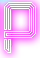# Product

#### Statement

Let P be the product of all numbers from 1 to N. Write a program that calculates the last non-zero digit of number P.

#### Input:

The first and only line of standard input contains the number N.

#### Output:

The standard output should contains the last non-zero digit of number P.

• 1 ≤ N ≤ 104

#### Example input:

``10``

#### Example output:

``8``

• 0.1 seconds
• 16MB

#### Explanation:

1 * 2 * 3 * 4 * 5 * 6 * 7 * 8 * 9 * 10 = 3628800 and the last non-zero digit is 8.

Problem source: Z-Trening